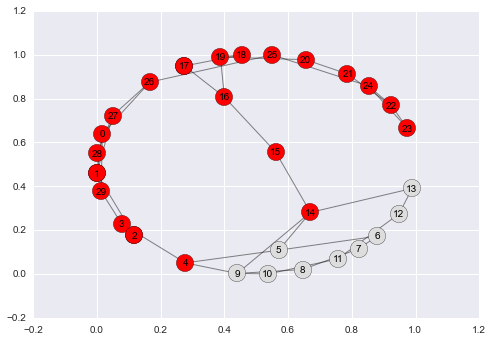# Basic Networks¶

In this notebook, we'll explore one of the most popular types of environments - a network. In the cells below, we'll be creating a small-world network and simulating a disease outbreak.

### Imports¶

In the import section below, you'll see an important new addition: networkx. We'll be using networkx as our preferred package for creating and managing network objects; we'll include links to more info and documentation in later cells.

In :
%matplotlib inline

# Imports
import networkx as nx
import numpy
import matplotlib.pyplot as plt
import pandas

import seaborn; seaborn.set()

# Import widget methods
from IPython.html.widgets import *


## Initializing a network¶

In some problems, we can define our own rules to "grow" or "generate" a network. However, for many problems, we may want to re-use an existing methodology. networkx comes with a suite of methods to "sample" random graphs, such as:

• Trees, e.g., balanced trees (networkx.balanced_tree)
• Erdos-Renyi graphs (networkx.erdos_renyi_graph)
• Watts-Strogatz small-world graphs (networkx.watts_strogatz_graph)
• Bipartite graphs

For a full list, see this page on Graph Generators in the networkx documentation.

In the sample below, we'll create a Newman-Watts-Strogatz small-world graph and print some basic info about the graph.

In :
# Create a random graph
nodes = 30
edges = 2
prob_out = 0.2
g = nx.newman_watts_strogatz_graph(nodes, edges, prob_out)
print((g.number_of_nodes(), g.number_of_edges()))

(30, 38)


## Visualizing a network¶

As humans, we are wired for visuals; for networks, visualizations can provide an important first characterization that isn't apparent from simple statistics.

In the cell below, we show how to calculate a "layout" for a network and visualize it using networkx.

For more examples, see the Drawing Graphs section of the NetworkX tutorial.

In :
# Draw the random graph
g_layout = nx.spring_layout(g, iterations=1000)
nx.draw_networkx(g, pos=g_layout, node_color='#dddddd')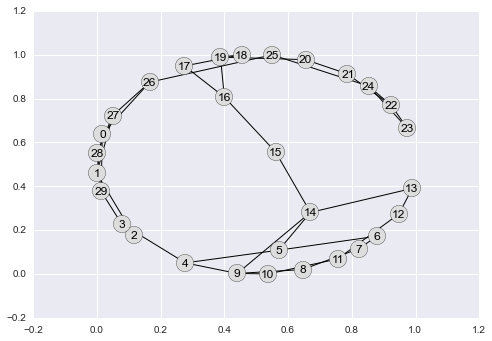## Outbreak in a network¶

Next, we'll simulate a very simple outbreak in a network. Our disease will start by infecting a random patient, then pass with probability 1 to any connected individuals.

To find the "connected" individuals, we'll need to learn how to find the neighbors of a node. NetworkX makes this easy.

In :
# Let's pick a node at random to infect
patient_zero = numpy.random.choice(g.nodes())
patient_zero

healthy_nodes = g.nodes()
healthy_nodes.remove(patient_zero)

In :
# Now we can visualize the infected node's position
f = plt.figure()
nx.draw_networkx_nodes(g, g_layout,
nodelist=[patient_zero],
node_color='red')

nx.draw_networkx_nodes(g, g_layout,
nodelist=healthy_nodes,
node_color='#dddddd')

nx.draw_networkx_edges(g, g_layout,
width=1.0,
alpha=0.5,
edge_color='#111111')

_ = nx.draw_networkx_labels(g, g_layout,
dict(zip(g.nodes(), g.nodes())),
font_size=10)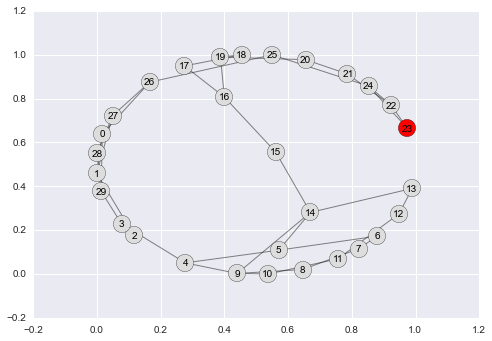## First model step¶

In our simple disease outbreak model, each step of the model consists of the following:

• for each infected individual, find their neighbors
• infect all neighbors

For our first step, we start with a single infected person; in subsequent steps, we need to use a for loop to handle all currently infected persons.

In :
# Find patient zero's neighbors
neighbors = g.neighbors(patient_zero)
neighbors

# Let's infect all of his neighbors!
infected_patients = [patient_zero]
infected_patients.extend(neighbors)
infected_patients

# Remove the infected from healthy nodes
healthy_nodes = [node for node in healthy_nodes if node not in infected_patients]

In :
# Now we can visualize the infected node's position
f = plt.figure()
nx.draw_networkx_nodes(g, g_layout,
nodelist=infected_patients,
node_color='red')

nx.draw_networkx_nodes(g, g_layout,
nodelist=healthy_nodes,
node_color='#dddddd')

nx.draw_networkx_edges(g, g_layout,
width=1.0,
alpha=0.5,
edge_color='#111111')

_ = nx.draw_networkx_labels(g, g_layout,
dict(zip(g.nodes(), g.nodes())),
font_size=10)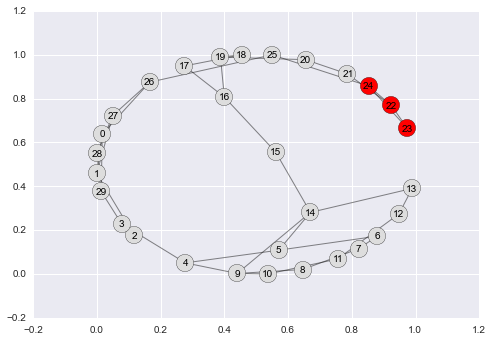## Second model step¶

As mentioned above, we now need to use a for loop over all individuals.

In :
# Now let's infect the neighbors of all infected patients!
newly_infected = []
for infected_patient in infected_patients:
# Find patient zero's neighbors
neighbors = [neighbor for neighbor in g.neighbors(infected_patient) if neighbor not in infected_patients]
newly_infected.extend(neighbors)

newly_infected

Out:
[25, 21]
In :
# Update infected and healthy
infected_patients.extend(newly_infected)

# Remove the infected from healthy nodes
healthy_nodes = [node for node in healthy_nodes if node not in infected_patients]

In :
# Now we can visualize the infected node's position
f = plt.figure()
nx.draw_networkx_nodes(g, g_layout,
nodelist=infected_patients,
node_color='red')

nx.draw_networkx_nodes(g, g_layout,
nodelist=healthy_nodes,
node_color='#dddddd')

nx.draw_networkx_edges(g, g_layout,
width=1.0,
alpha=0.5,
edge_color='#111111')

_ = nx.draw_networkx_labels(g, g_layout,
dict(zip(g.nodes(), g.nodes())),
font_size=10)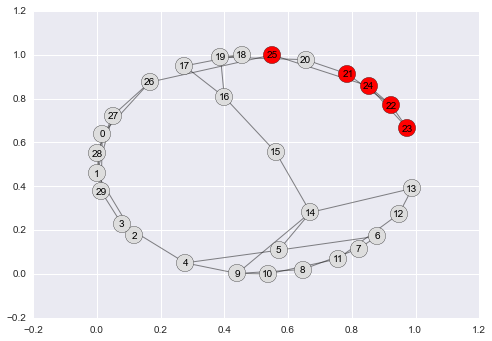## Running a few more steps¶

Let's wrap our model step method in yet another for loop, allowing us to simulate multiple steps of the model at once.

In :
# Now let's infect the neighbors of all infected patients!
model_steps = 5

# Iterate over steps
for i in range(model_steps):
# Iterate over infected
newly_infected = []
for infected_patient in infected_patients:
# Find patient neighbors and infect
neighbors = [neighbor for neighbor in g.neighbors(infected_patient) if neighbor not in infected_patients]
newly_infected.extend(neighbors)

# Remove the infected from healthy nodes
infected_patients.extend(newly_infected)
healthy_nodes = [node for node in healthy_nodes if node not in infected_patients]

# Now we can visualize the infected node's position
f = plt.figure()
nx.draw_networkx_nodes(g, g_layout,
nodelist=infected_patients,
node_color='red')

nx.draw_networkx_nodes(g, g_layout,
nodelist=healthy_nodes,
node_color='#dddddd')

nx.draw_networkx_edges(g, g_layout,
width=1.0,
alpha=0.5,
edge_color='#111111')

_ = nx.draw_networkx_labels(g, g_layout,
dict(zip(g.nodes(), g.nodes())),
font_size=10)